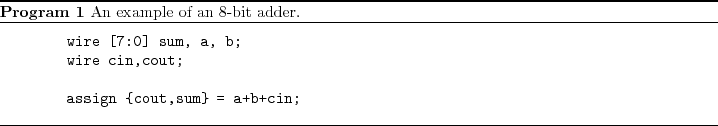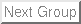Up: Lab 5: Arithmetic Logic Previous: 2 Lab

Subsections

# 3 Supplementary Material

## 3.1 Verilog

### 3.1.1 Parameters

Parameters are constants and not variables.

parameter num = 8;

### 3.1.2 Operators

#### 3.1.2.1 ?:Construct

assign y = sel?a:b;

If sel is true, then y is assigned a, else it is assigned b.

#### 3.1.2.2 Concatenations

In Verilog it is possible to concatenate bits using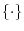.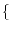a, b, c, a, b, c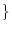is equivalent to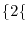a, b, c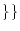#### 3.1.2.3 Comparison Operators

assign y = a>b?a:b;

assign y to a if a>b and assign it to b otherwise. Table 2 shows a list of comparison operators.

 Operator Description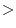greater than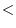less than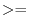greater than or equal to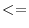less than or equal to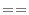equality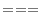equality including x and z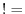inequality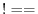inequality including x and z

• forandthe result is x, if either operand contains an x or z.

#### 3.1.2.4 Logical Operators

Table 3 shows a list of logical operators.

 Operator Description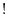logical negation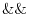logical AND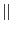logical OR

• Evaluation is performed left to right.
• x if any of the operands has unknown x bits.

#### 3.1.2.5 Binary Arithmetic Operators

Table 4 shows a list of arithmetic operators.

 Operator Description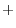addition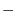subtraction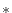multiplication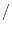division (truncates any fractional part)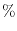equality

#### 3.1.2.6 Unary Arithmetic Operators

Table 5 shows a list of unary arithmetic operators.

 Operator DescriptionChange the sign of the operand

#### 3.1.2.7 Bitwise Operators

Table 6 shows a list of bitwise operators.

 Operator Description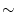Bitwise negation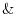Bitwise AND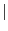Bitwise OR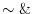Bitwise NAND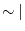Bitwise OR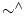or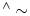Equivalence

#### 3.1.2.8 Unary Reduction Operators

Table 7 shows a list of unary reduction operators. They produce a single bit result by applying the operator to all of the pits of the operand.

 Operator DescriptionBitwise negationBitwise ANDBitwise ORBitwise NANDBitwise ORorEquivalence

#### 3.1.2.9 Shift Operators

Table 8 shows a list of shift operators.

 Operator Description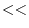left shift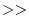right shift

• Left operand is shifted by the number of bit positions given by the right operand.
• Zeros are used to fill vacated bit positions.

#### 3.1.2.10 Operator Precedence Rule

Table 9 shows a list operator precedence rules.

 !,Highest Precedence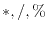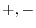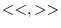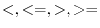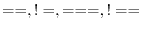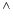,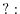Lowest Precedence